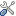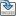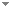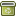# Chaitin’s ω as a continuous function

Journal of Symbolic Logic 85 (1):486-510 (2020)

 Authors Abstract We prove that the continuous function${\rm{\hat \Omega }}:2^\omega \to$ that is defined via$X \mapsto \mathop \sum \limits_n 2^{ - K\left}$ for all $X \in {2^\omega }$ is differentiable exactly at the Martin-Löf random reals with the derivative having value 0; that it is nowhere monotonic; and that $\mathop \smallint \nolimits _0^1{\rm{\hat{\Omega }}}\left\,{\rm{d}}X$ is a left-c.e. $wtt$-complete real having effective Hausdorff dimension ${1 / 2}$.We further investigate the algorithmic properties of ${\rm{\hat{\Omega }}}$. For example, we show that the maximal value of ${\rm{\hat{\Omega }}}$ must be random, the minimal value must be Turing complete, and that ${\rm{\hat{\Omega }}}\left \oplus X{ \ge _T}\emptyset \prime$ for every X. We also obtain some machine-dependent results, including that for every $\varepsilon > 0$, there is a universal machine V such that ${{\rm{\hat{\Omega }}}_V}$ maps every real X having effective Hausdorff dimension greater than ε to a real of effective Hausdorff dimension 0 with the property that $X{ \le _{tt}}{{\rm{\hat{\Omega }}}_V}\left$; and that there is a real X and a universal machine V such that ${{\rm{\Omega }}_V}\left$ is rational. Keywords No keywords specified (fix it) Categories (categorize this paper) DOI 10.1017/jsl.2019.60 OptionsEdit this recordMark as duplicateExport citationFind it on ScholarRequest removal from indexRevision history

PhilArchive copy

Upload a copy of this paper     Check publisher's policy     Papers currently archived: 71,259

Setup an account with your affiliations in order to access resources via your University's proxy server
Configure custom proxy (use this if your affiliation does not provide a proxy)

## References found in this work BETA

No references found.

## Citations of this work BETA

No citations found.

## Similar books and articles

Degrees of Unsolvability of Continuous Functions.Joseph S. Miller - 2004 - Journal of Symbolic Logic 69 (2):555-584.
Lp -Computability.Ning Zhong & Bing-Yu Zhang - 1999 - Mathematical Logic Quarterly 45 (4):449-456.
Realizability Models Refuting Ishiharaʼs Boundedness Principle.Peter Lietz & Thomas Streicher - 2012 - Annals of Pure and Applied Logic 163 (12):1803-1807.
A Very Discontinuous Borel Function.Juris Steprāns - 1993 - Journal of Symbolic Logic 58 (4):1268 - 1283.
A Very Discontinuous Borel Function.Juris Steprans - 1994 - Journal of Symbolic Logic 59 (4):1268-1283.
Derivatives of Computable Functions.Ning Zhong - 1998 - Mathematical Logic Quarterly 44 (3):304-316.
Ω in Number Theory.Toby Ord - 2007 - In C. S. Calude (ed.), Randomness and Complexity, from Leibniz to Chaitin. World Scientific. pp. 161-173.
Leibniz, Complexidade E Incompletude.Gregory J. Chaitin & Virginia Maria F. Gonçalves Chaitin - 2016 - Veritas – Revista de Filosofia da Pucrs 61 (2):295-305.